DOC HOME SITE MAP MAN PAGES GNU INFO SEARCH PRINT BOOK
 Home | All Classes | Main Classes | Annotated | Grouped Classes | Functions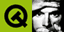# QRegion Class Reference

The QRegion class specifies a clip region for a painter. More...

#include <qregion.h>

## Public Members

• enum RegionType { Rectangle, Ellipse }
• QRegion ()
• QRegion ( int x, int y, int w, int h, RegionType t = Rectangle )
• QRegion ( const QRect & r, RegionType t = Rectangle )
• QRegion ( const QPointArray & a, bool winding = FALSE )
• QRegion ( const QRegion & r )
• QRegion ( const QBitmap & bm )
• ~QRegion ()
• QRegion & operator= ( const QRegion & r )
• bool isNull () const
• bool isEmpty () const
• bool contains ( const QPoint & p ) const
• bool contains ( const QRect & r ) const
• void translate ( int dx, int dy )
• QRegion unite ( const QRegion & r ) const
• QRegion intersect ( const QRegion & r ) const
• QRegion subtract ( const QRegion & r ) const
• QRegion eor ( const QRegion & r ) const
• QRect boundingRect () const
• QMemArray<QRect> rects () const
• const QRegion operator| ( const QRegion & r ) const
• const QRegion operator+ ( const QRegion & r ) const
• const QRegion operator& ( const QRegion & r ) const
• const QRegion operator- ( const QRegion & r ) const
• const QRegion operator^ ( const QRegion & r ) const
• QRegion & operator|= ( const QRegion & r )
• QRegion & operator+= ( const QRegion & r )
• QRegion & operator&= ( const QRegion & r )
• QRegion & operator-= ( const QRegion & r )
• QRegion & operator^= ( const QRegion & r )
• bool operator== ( const QRegion & r ) const
• bool operator!= ( const QRegion & r ) const
• HRGN handle () const

## Related Functions

• QDataStream & operator<< ( QDataStream & s, const QRegion & r )
• QDataStream & operator>> ( QDataStream & s, QRegion & r )

## Detailed Description

The QRegion class specifies a clip region for a painter.

QRegion is used with QPainter::setClipRegion() to limit the paint area to what needs to be painted. There is also a QWidget::repaint() that takes a QRegion parameter. QRegion is the best tool for reducing flicker.

A region can be created from a rectangle, an ellipse, a polygon or a bitmap. Complex regions may be created by combining simple regions using unite(), intersect(), subtract() or eor() (exclusive or). You can move a region using translate().

You can test whether a region isNull(), isEmpty() or if it contains() a QPoint or QRect. The bounding rectangle is given by boundingRect().

The function rects() gives a decomposition of the region into rectangles.

Example of using complex regions:

```        void MyWidget::paintEvent( QPaintEvent * )
{
QPainter p;                         // our painter
QRegion r1( QRect(100,100,200,80),  // r1 = elliptic region
QRegion::Ellipse );
QRegion r2( QRect(100,120,90,30) ); // r2 = rectangular region
QRegion r3 = r1.intersect( r2 );    // r3 = intersection
p.begin( this );                    // start painting widget
p.setClipRegion( r3 );              // set clip region
...                                 // paint clipped graphics
p.end();                            // painting done
}
```

QRegion is an implicitly shared class.

Warning: Due to window system limitations, the whole coordinate space for a region is limited to the points between -32767 and 32767 on Mac OS X and Windows 95/98/ME.

## Member Type Documentation

### QRegion::RegionType

Specifies the shape of the region to be created.

• QRegion::Rectangle - the region covers the entire rectangle.
• QRegion::Ellipse - the region is an ellipse inside the rectangle.

## Member Function Documentation

### QRegion::QRegion ()

Constructs a null region.

### QRegion::QRegion ( int x, int y, int w, int h, RegionType t = Rectangle )

Constructs a rectangular or elliptic region.

If t is Rectangle, the region is the filled rectangle (x, y, w, h). If t is Ellipse, the region is the filled ellipse with center at (x + w / 2, y + h / 2) and size (w ,h ).

### QRegion::QRegion ( const QRect & r, RegionType t = Rectangle )

This is an overloaded member function, provided for convenience. It behaves essentially like the above function.

Create a region based on the rectange r with region type t.

If the rectangle is invalid a null region will be created.

### QRegion::QRegion ( const QPointArray & a, bool winding = FALSE )

Constructs a polygon region from the point array a.

If winding is TRUE, the polygon region is filled using the winding algorithm, otherwise the default even-odd fill algorithm is used.

This constructor may create complex regions that will slow down painting when used.

### QRegion::QRegion ( const QRegion & r )

Constructs a new region which is equal to region r.

### QRegion::QRegion ( const QBitmap & bm )

Constructs a region from the bitmap bm.

The resulting region consists of the pixels in bitmap bm that are color1, as if each pixel was a 1 by 1 rectangle.

This constructor may create complex regions that will slow down painting when used. Note that drawing masked pixmaps can be done much faster using QPixmap::setMask().

### QRegion::~QRegion ()

Destroys the region.

### QRectQRegion::boundingRect () const

Returns the bounding rectangle of this region. An empty region gives a rectangle that is QRect::isNull().

### bool QRegion::contains ( const QPoint & p ) const

Returns TRUE if the region contains the point p; otherwise returns FALSE.

### bool QRegion::contains ( const QRect & r ) const

This is an overloaded member function, provided for convenience. It behaves essentially like the above function.

Returns TRUE if the region overlaps the rectangle r; otherwise returns FALSE.

### QRegionQRegion::eor ( const QRegion & r ) const

Returns a region which is the exclusive or (XOR) of this region and r.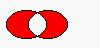The figure shows the exclusive or of two elliptical regions.

### HRGN QRegion::handle () const

Returns the region's handle.

### QRegionQRegion::intersect ( const QRegion & r ) const

Returns a region which is the intersection of this region and r.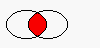The figure shows the intersection of two elliptical regions.

### bool QRegion::isEmpty () const

Returns TRUE if the region is empty; otherwise returns FALSE. An empty region is a region that contains no points.

Example:

```        QRegion r1( 10, 10, 20, 20 );
QRegion r2( 40, 40, 20, 20 );
QRegion r3;
r1.isNull();             // FALSE
r1.isEmpty();            // FALSE
r3.isNull();             // TRUE
r3.isEmpty();            // TRUE
r3 = r1.intersect( r2 ); // r3 = intersection of r1 and r2
r3.isNull();             // FALSE
r3.isEmpty();            // TRUE
r3 = r1.unite( r2 );     // r3 = union of r1 and r2
r3.isNull();             // FALSE
r3.isEmpty();            // FALSE
```

### bool QRegion::isNull () const

Returns TRUE if the region is a null region; otherwise returns FALSE.

A null region is a region that has not been initialized. A null region is always empty.

### bool QRegion::operator!= ( const QRegion & r ) const

Returns TRUE if the region is different from r; otherwise returns FALSE.

### const QRegionQRegion::operator& ( const QRegion & r ) const

Applies the intersect() function to this region and r. r1&r2 is equivalent to r1.intersect(r2)

### QRegion & QRegion::operator&= ( const QRegion & r )

Applies the intersect() function to this region and r and assigns the result to this region. r1&=r2 is equivalent to r1=r1.intersect(r2)

### const QRegionQRegion::operator+ ( const QRegion & r ) const

Applies the unite() function to this region and r. r1+r2 is equivalent to r1.unite(r2)

### QRegion & QRegion::operator+= ( const QRegion & r )

Applies the unite() function to this region and r and assigns the result to this region. r1+=r2 is equivalent to r1=r1.unite(r2)

### const QRegionQRegion::operator- ( const QRegion & r ) const

Applies the subtract() function to this region and r. r1-r2 is equivalent to r1.subtract(r2)

### QRegion & QRegion::operator-= ( const QRegion & r )

Applies the subtract() function to this region and r and assigns the result to this region. r1-=r2 is equivalent to r1=r1.subtract(r2)

### QRegion & QRegion::operator= ( const QRegion & r )

Assigns r to this region and returns a reference to the region.

### bool QRegion::operator== ( const QRegion & r ) const

Returns TRUE if the region is equal to r; otherwise returns FALSE.

### const QRegionQRegion::operator^ ( const QRegion & r ) const

Applies the eor() function to this region and r. r1^r2 is equivalent to r1.eor(r2)

### QRegion & QRegion::operator^= ( const QRegion & r )

Applies the eor() function to this region and r and assigns the result to this region. r1^=r2 is equivalent to r1=r1.eor(r2)

### const QRegionQRegion::operator| ( const QRegion & r ) const

Applies the unite() function to this region and r. r1|r2 is equivalent to r1.unite(r2)

### QRegion & QRegion::operator|= ( const QRegion & r )

Applies the unite() function to this region and r and assigns the result to this region. r1|=r2 is equivalent to r1=r1.unite(r2)

### QMemArray<QRect> QRegion::rects () const

Returns an array of non-overlapping rectangles that make up the region.

The union of all the rectangles is equal to the original region.

### QRegionQRegion::subtract ( const QRegion & r ) const

Returns a region which is r subtracted from this region.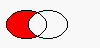The figure shows the result when the ellipse on the right is subtracted from the ellipse on the left. (left-right )

### void QRegion::translate ( int dx, int dy )

Translates (moves) the region dx along the X axis and dy along the Y axis.

### QRegionQRegion::unite ( const QRegion & r ) const

Returns a region which is the union of this region and r.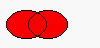The figure shows the union of two elliptical regions.

## Related Functions

### QDataStream & operator<< ( QDataStream & s, const QRegion & r )

Writes the region r to the stream s and returns a reference to the stream.

See also Format of the QDataStream operators.

### QDataStream & operator>> ( QDataStream & s, QRegion & r )

Reads a region from the stream s into r and returns a reference to the stream.

See also Format of the QDataStream operators.